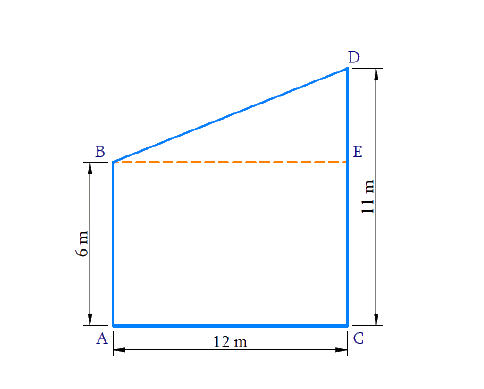# Ex.6.5 Q12 Triangles Solution - NCERT Maths Class 10

## Question

Two poles of heights $$6\;\rm{}m$$ and $$11\;\rm{}m$$ stand on plane ground. If the distance between the feet of the poles is $$12\;\rm{}m$$, find the distance between their tops.

DiagramVideo Solution
Triangles
Ex 6.5 | Question 12

## Text Solution

Reasoning:

In a right triangle, the square of the hypotenuse is equal to the sum of the squares of the other two sides.

Steps:

$$AB$$ is the height of one pole $$= 6\;\rm{}m$$

$$CD$$ is the height of another pole $$= 11\,\rm{}m$$

$$AC$$ is the distance between two poles at bottom $$= 12\rm{}\,m$$

$$BD$$ is the distance between the tops of the poles $$=\,?$$

Draw $$B E \| A C$$

Now consider,

In $$\Delta B E D$$

\begin{align}\angle B E D&=90^{\circ} \\ {BE}={AC}&=12 \mathrm{m}\\ {DE}&={CD}-\mathrm{CE} \\ {DE}&=11-6=5 \mathrm{cm}\\\end{align}

Now,

\begin{align} B D^{2} &=B E^{2}+D E^{2}\\ & [ \text {Pythagoras } \text{ theorem} ] \\\\ &=12^{2}+5^{2} \\ &=144+25 \\ B D^{2} &=169 \\ B D &=13 \mathrm{m} \end{align}

The distance between the tops of poles $$=13\rm{}\,m$$

Learn from the best math teachers and top your exams

• Live one on one classroom and doubt clearing
• Practice worksheets in and after class for conceptual clarity
• Personalized curriculum to keep up with school